Superconductivity has been the focus of enormous research efforts since its discovery more than a century ago.

Yet, some features of this unique phenomenon remain poorly understood. The prime among these is the connection between chemical/structural properties of materials and the superconductivity phenomenon.

Knowing the critical temperature of a superconductor is crucial because at that temperature the material exhibits zero electrical resistance.

For this study, we model superconductors’ critical temperatures from an extensive data set of the chemical properties of 21263 superconductors.

### Contents

We solve this example with the data science and machine learning platform Neural Designer. To follow it step by step, you can use the free trial.

## 1. Application type

This is an approximation project since the variable to be predicted is continuous (Critical Temperature).

This study uses a data-driven approach to create a statistical model that predicts Tc based on its chemical formula.

## 2. Data set

The first step is to prepare the data set, which is the source of information for the approximation problem. It is composed of:

• Data source.
• Variables.
• Instances.

The file superconductor.csv contains the data for this example. Here, the number of variables (columns) is 82, and the number of instances (rows) is 21263.

### Variables

In that way, this problem has the following variables:

• atomic_mass: Total proton and neutron rest masses, in Atomic Mass Units (AMU).
• first_ionization_energy: Energy required to remove a valence electron, in kilo-Joules per mole (kJ/mol).
• density: Density at standard temperature and pressure, in kilograms per meter cubed (kg/m3).
• electron_affinity:  Energy required to add an electron to a neutral atom, in kilo-Joules per mole (kJ/mol).
• fusion_heat: Energy to change from solid to liquid without temperature change, in kilo-joules per mole (kJ/mol).
• thermal_conductivity: Thermal conductivity coefficient k, in watts per meter-kelvin (W/(m ?? K)).
• valence: Typical number of chemical bonds formed by the element, no units.
• critical_temperature: Superconductor critical temperature, in Kelvin.

These are the main variables of this study. They correspond to the chemical properties of each compound in the following dataset: chemical_compounds.csv.

Statistics of each variable include mean, weighted mean, geometric mean, weighted geometric mean, entropy, weighted entropy, standard, weighted standard, range, and weighted range.

The ratios of the elements in the material are used to define features:
$$p_{i}=\frac{j}{\sum_{i=1}^{n} j}$$
Where $j$ is the proportion of an element in the compound.

The fractions of total thermal conductivities are used as well:

$$w_{i}=\frac{t_{i}}{\sum_{i=1}^{n} t_{i}}$$
Where $t_{i}$ are the thermal conductivity coefficients.

We will also need intermediate values for calculating features:

$$A_{i}=\frac{p_{i}w_{i}}{\sum_{i=1}^{n} p_{i}w_{i}}$$

The following table summarizes the procedure for feature extraction from the material’s chemical formula.

Feature & description Formula
Mean $$\mu=\sum_{i=1}^{n} \frac{t_{i}}{i}$$
Weighted mean $$\nu=\sum_{i=1}^{n} p_{i} t_{i}$$
Geometric mean $$\sqrt{\sum_{i=1}^{n} t_{i}}$$
Weighted geometric mean $$\sum_{i=1}^{n} t_{i}^{p_{i}}$$
Entropy $$-\sum_{i=1}^{n} w_{i} ln w_{i}$$
Weighted entropy $$-\sum_{i=1}^{n} A_{i} ln A_{i}$$
Range $$t_{(max)} – t_{(min)}$$
Weighted range $$p(t_{(max)})t_{(max)} – p(t_{(min)})t_{(min)}$$
Standard deviation $$\left[\frac{1}{2}\left(\sum_{i=1}^{n} (t_{i}-\mu)^{2}\right)\right]^{\frac{1}{2}}$$
Weighted standard deviation $$\left[\sum_{i=1}^{n} p_{i}(t_{i}-\nu)^{2}\right]^{\frac{1}{2}}$$

For instance, for the chemical compound Re7Zr1 with these Rhenium and Zirconium’s thermal conductivity coefficients: $t_{1} = 48\,\,W/(mK)$ and $t_{2} = 23\,\,W/(mK)$, respectively.

We can calculate features like the weighted geometric mean and obtain a value of $43.21$

They are divided randomly into trainingselection, and testing subsets, containing 60%, 20%, and 20% of the instances, respectively. More specifically, 12759 samples are used here for training, 4252 for validation, and 4252 for testing.

Once all the data set information has been established, we will perform some analytics to check the quality of the data.

### Data distribution

For instance, we can calculate the data distribution. The following figure depicts the histogram for the target variable.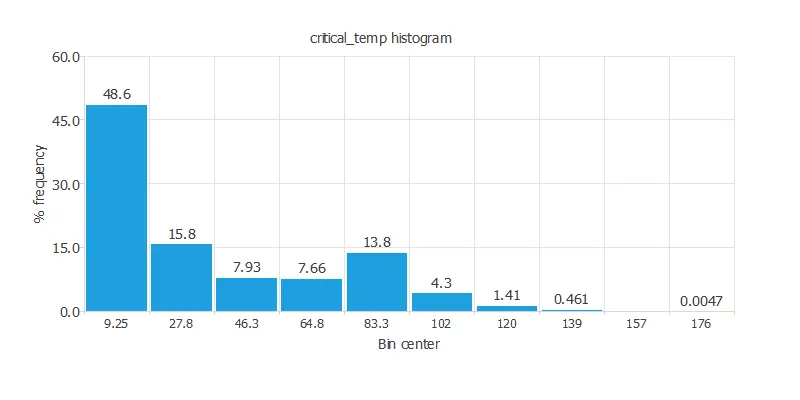The above graph shows more chemical compounds with low critical temperatures.

This could be explained because finding a superconductor with a relatively high critical temperature is difficult. To find superconductor properties, such as current conductivity with zero resistance, we have to reduce a lot the material temperature.

### Inputs-targets correlations

The next figure depicts inputs-targets correlations. This might help us see the different inputs’ influence on the critical temperature.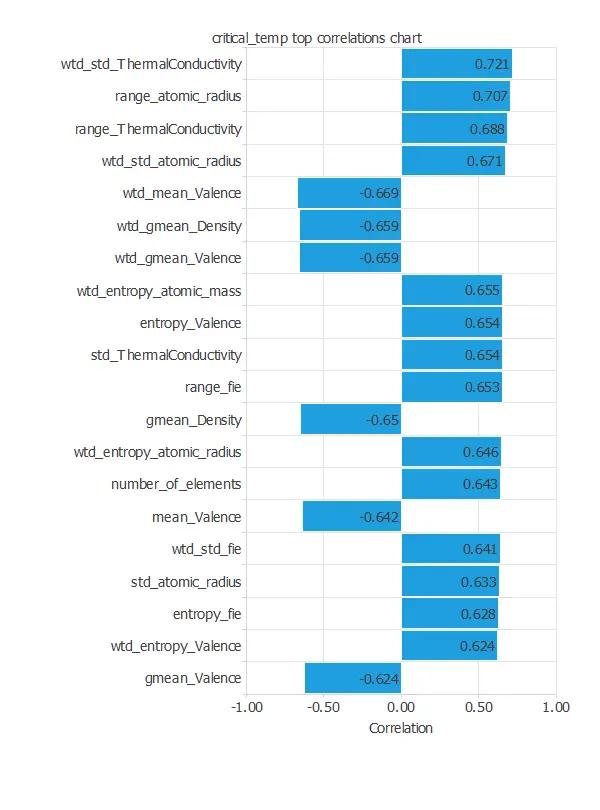As there are so many input variables, the chart shows the top 20.

We can also plot a scatter chart with the critical temperature versus the weighted mean valence.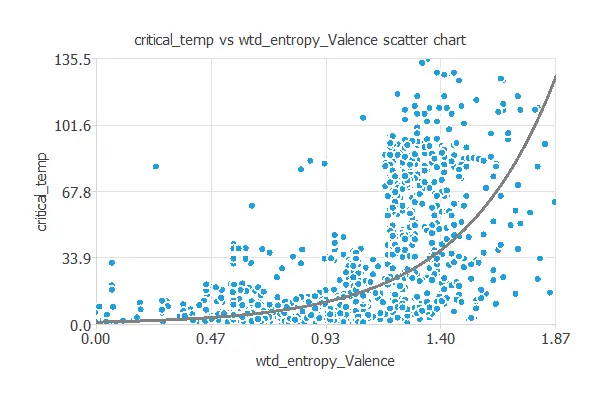As we can see, the critical temperature decreases when we increase the weighted mean valence logarithmically.

## 3. Neural network

The neural network will output the critical temperature as a function of different chemical properties.

For this approximation example, the neural network is composed of:

• Scaling layer.
• Perceptron layers.
• Unscaling layer.

The scaling layer transforms the original inputs to normalized values. Here, the Minimum-Maximum deviation scaling method is set so that the input values have a minimum of -1 and a maximum of +1.

Here two perceptron layers are added to the neural network. This number of layers is enough for most applications. The first layer has 81 inputs and 3 neurons. The second layer has 3 inputs and 1 neuron.

The unscaling layer transforms the normalized values from the neural network into the original outputs. Here, the Minimum-Maximum deviation scaling method and mean and standard deviation unscaling method will also be used.

The figure below shows the final architecture for the neural network.## 4. Training strategy

The next step is selecting an appropriate training strategy to define what the neural network will learn. A general training strategy is composed of two concepts:

• A loss index.
• An optimization algorithm.

The loss index chosen is the normalized squared error with L2 regularization. This loss index is the default in approximation applications.

The optimization algorithm chosen is the quasi-Newton method. This optimization algorithm is the default for medium-sized applications like this one.

Once the strategy has been set, we can train the neural network. The following chart shows how the training (blue) and selection (orange) errors decrease with the training epoch during the training process.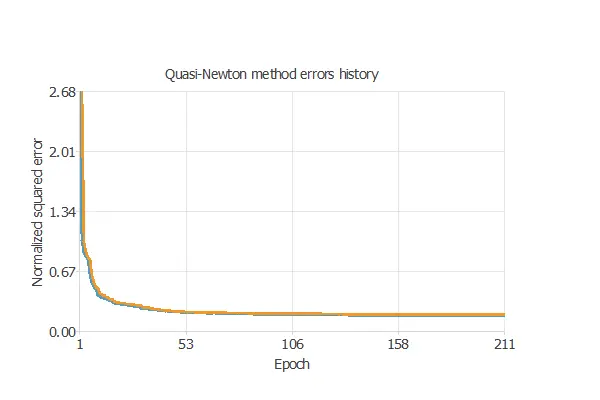The most crucial training result is the final selection error. Indeed, this is a measure of the generalization capabilities of the neural network. Here, the final selection error is selection error = 0.178 NSE.

## 5. Model selection

The objective of model selection is to find the network architecture with the best generalization properties. We want to improve the final selection error obtained before (0.178 NSE).

The best selection error is achieved by using a model whose complexity is the most appropriate to produce a good data fit. Order selection algorithms are responsible for finding the optimal number of perceptrons in the neural network.

The final selection error takes a minimum value at some point. Here, the optimal number of neurons is 10, corresponding to a 0.164 selection error.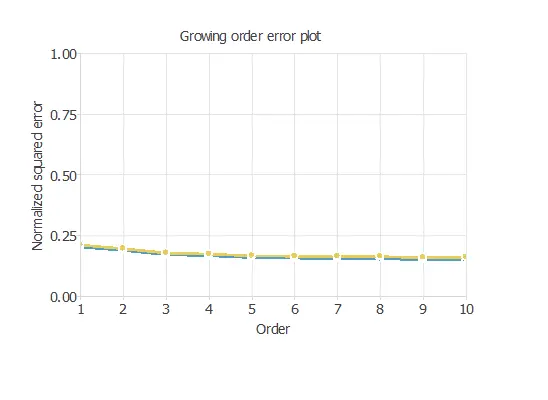The above chart shows the error history for the different subsets during the growing neurons selection process. The blue line represents the training error, and the yellow line symbolizes the selection error.

## 6. Testing analysis

The objective of the testing analysis is to validate the generalization performance of the trained neural network. The testing compares the values provided by this technique to the observed values.

A standard testing technique in approximation problems is to perform a linear regression analysis between the predicted and the real values, using an independent testing set. The following figure illustrates a graphical output provided by this testing analysis.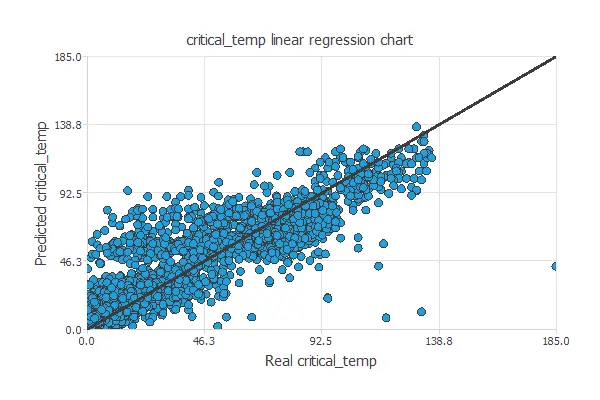From the above chart, we can see that the neural network is predicting well the entire range of the critical temperature data. The correlation value is R2 = 0.911, which is very close to 1.

## 7. Model deployment

The model is now ready to estimate the critical temperature of a specific chemical compound.

We can plot a neural network’s directional output to see how the emissions vary with a given input for all other fixed inputs. The following plot shows the critical temperature as a function of the geometric mean valence through the following point: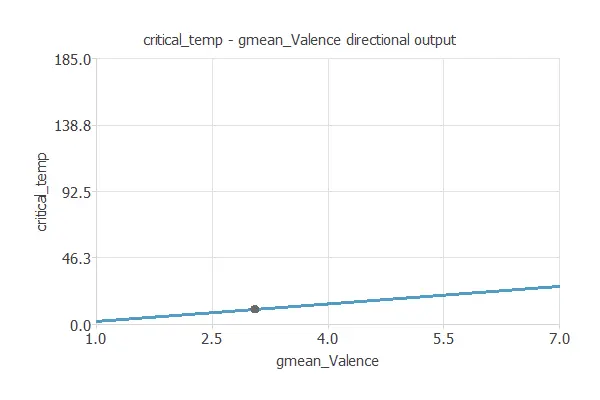For this study, it is important to mention other valuable tasks of the Model Deployment Tool we are referring to: Calculate Outputs.

We could think about creating a semiconductor with specific chemical quantities. With this tool, we can select the inputs and calculate the optimal superconductor critical temperature for our desired purpose.

The superconductor.py contains the Python code to calculate a compound critical temperature.

## Conclusions

In this post, we build a machine learning model to estimate the superconducting critical temperature as a function of the features extracted from the superconductor’s chemical formula.

These features include atomic radius, valence, electron affinity, atomic mass, etc.